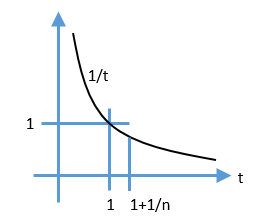# How does that limit for e work?

You may have seen the following equation:
\begin{align}
\lim_{n \rightarrow \infty} \left( 1 +\frac{1}{n} \right)^n = \mathrm{e}.
\end{align}

which expresses the fundamental constant e as a limit. Wondering how that can be proven?

Consider this graph:One key fact we will use is

\begin{align}
\int_1^{x} \, \frac{1}{t} \mathrm{d}t = \ln x
\end{align}

Looking at the graph, you can see that the area under the $$\frac{1}{t}$$ curve on the interval $$(1,1+\frac{1}{n})$$ is bounded by

\begin{align}
\frac{1}{n}\left( \frac{1}{1+\frac{1}{n}} \right) \leq \int_1^{1+\frac{1}{n}} \, \frac{1}{t} \mathrm{d}t \leq \frac{1}{n} \left( 1 \right)
\end{align}

Using the definition of $$\ln x$$ above, we have

\begin{align}
\frac{1}{1+n} \leq \ln \left( 1+\frac{1}{n} \right) \leq \frac{1}{n}
\end{align}

Raising $$\mathrm{e}$$ to the power of each term,

\begin{align}
\mathrm{e}^{(\frac{1}{1+n})} \leq 1+\frac{1}{n} \leq \mathrm{e}^{(\frac{1}{n})}
\end{align}

Raising each term to power $$n$$,
\begin{align}
\mathrm{e}^{(\frac{n}{1+n})} \leq \left( 1+\frac{1}{n} \right)^n \leq \mathrm{e}
\end{align}

As n approaches infinity, the left hand term approaches $$\mathrm{e}$$, therefore
\begin{align}
\lim_{n \rightarrow \infty} \left( 1 +\frac{1}{n} \right)^n = \mathrm{e}.
\end{align}

QED.